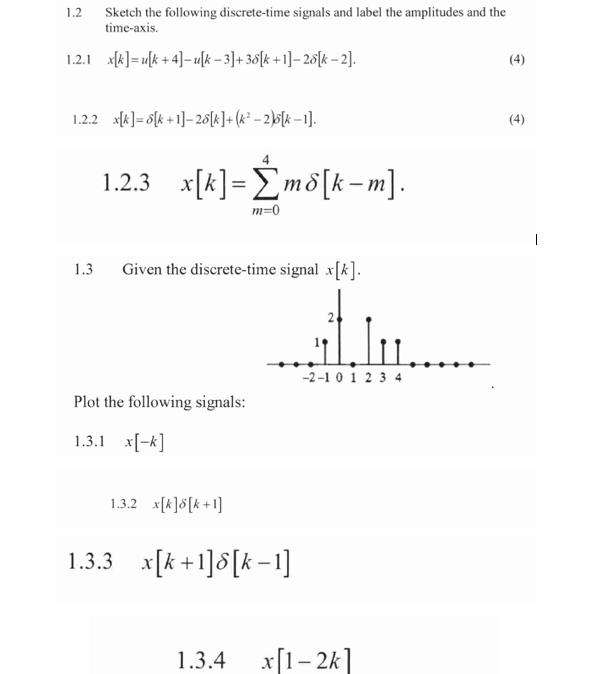Home / Expert Answers / Electrical Engineering / nbsp-1-2-sketch-the-following-discrete-time-signals-and-label-the-amplitudes-and-the-time-axis-pa121

# (Solved):   1.2 Sketch the following discrete-time signals and label the amplitudes and the time-axis. ...1.2 Sketch the following discrete-time signals and label the amplitudes and the time-axis. 1.2.1 $$x[k]=u[k+4]-u[k-3]+3 \delta[k+1]-2 \delta[k-2] .$$ 1.2.2 $$x[k]=\delta[k+1]-2 \delta[k]+\left(k^{2}-2\right) \delta[k-1]$$. 1.2.3 $$x[k]=\sum_{m=0}^{4} m \delta[k-m] .$$ $$1.3$$ Given the discrete-time signal $$x[k]$$. Plot the following signals: 1.3.1 $$x[-k]$$ 1.3.2 $$x[k] \delta[k+1]$$ 1.3.3 $$x[k+1] \delta[k-1]$$

We have an Answer from Expert